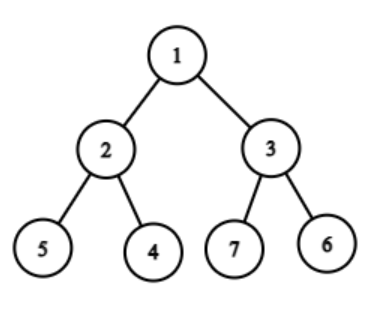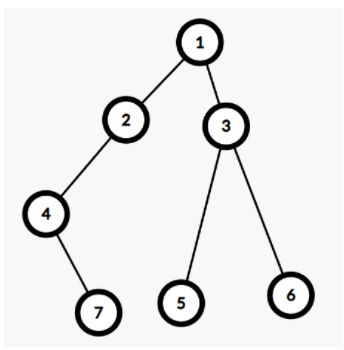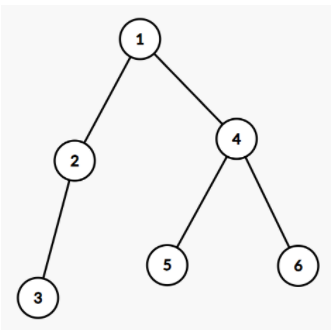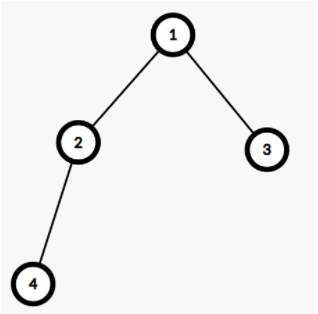6

Difficulty: EASYContributed By
Anish De
Avg. time to solve
15 min
Success Rate
80%

Problem Statement

#### A binary tree is a hierarchical data structure in which each node has at most two children.

##### Example:
``````Consider the given binary tree:
````````````For the given tree the leaf nodes are 5, 4, 7, 6. In this binary tree, the maximum sum path is 11(1 + 3 + 7). So the path 1 3 7 is printed.
``````
##### Input format :
``````The first line contains a single integer T representing the number of test cases.

The first line of each test case contains elements in the level order form(refer to the example below) (separated by space). If any node does not have a left or right child, take -1 in its place.
``````
##### Example:
``````Elements are in the level order form. The input consists of values of nodes separated by a single space in a single line. In case a node is null, we take -1 in its place.

For example, the input for the tree depicted in the below image would be :
1
2 3
4 -1 5 6
-1 7 -1 -1 -1 -1
-1 -1
````````````Explanation :
Level 1 :
The root node of the tree is 1

Level 2 :
Left child of 1 = 2
Right child of 1 = 3

Level 3 :
Left child of 2 = 4
Right child of 2 = null (-1)
Left child of 3 = 5
Right child of 3 = 6

Level 4 :
Left child of 4 = null (-1)
Right child of 4 = 7
Left child of 5 = null (-1)
Right child of 5 = null (-1)
Left child of 6 = null (-1)
Right child of 6 = null (-1)

Level 5 :
Left child of 7 = null (-1)
Right child of 7 = null (-1)

The first not-null node (of the previous level) is treated as the parent of the first two nodes of the current level. The second not-null node (of the previous level) is treated as the parent node for the next two nodes of the current level and so on.

The input ends when all nodes at the last level are null (-1).

Note: The above format was just to provide clarity on how the input is formed for a given tree.

The sequence will be put together in a single line separated by a single space. Hence, for the above-depicted tree, the input will be given as:

1 2 3 4 -1 5 6 -1 7 -1 -1 -1 -1 -1 -1
``````
##### Output format :
``````For each test case, print "True" if all the leaf nodes are at the same level otherwise print "False” (without quotes).

The output of each test case should be printed in a separate line.
``````
##### Note
``````You are not required to print anything, it has already been taken care of. Just implement the function.
``````
##### Constraints:
``````1 <= T <= 100
1 <= N <= 3000
-10^9 <= nodeVal <= 10^9

Where nodeVal denotes the value associated with the node.

Time Limit: 1 sec
``````
##### Sample Input 1:
``````2
1 2 4 3 -1 5 6 -1 -1 -1 -1 -1 -1
1 2 3 4 -1 -1 -1 -1 -1
``````
##### Sample Output 1:
``````1 4 6
1 2 4
``````
##### Explanation For Sample Output 1:
``````In the first test case, the leaves of the binary tree are 3 ( path-> 1 2 3 ) , 5 (path-> 1 4 5), and 6 (path-> 1 4 6). So, The maximum sum path is 11 (1+4+6). So 1 4 6 is printed.

Diagram of the Binary Tree given in the first test case of Sample Input 1.
````````````In the first test case, the leaves of the binary tree are 3 ( path-> 1 3 ) and 4 (path-> 1 2 4).
So, The maximum path is 7 (1+2+4). So 1 2 4 is printed.

Diagram of the Binary Tree given in the second test case of Sample Input 1.
``````##### Sample Input 2:
``````2
1 2 3 -1 -1 4 -1 -1 -1
1 2 -1 3 -1 4 -1 -1 -1
``````
##### Sample Output 2:
``````1 3 4
1 2 3 4
``````Console